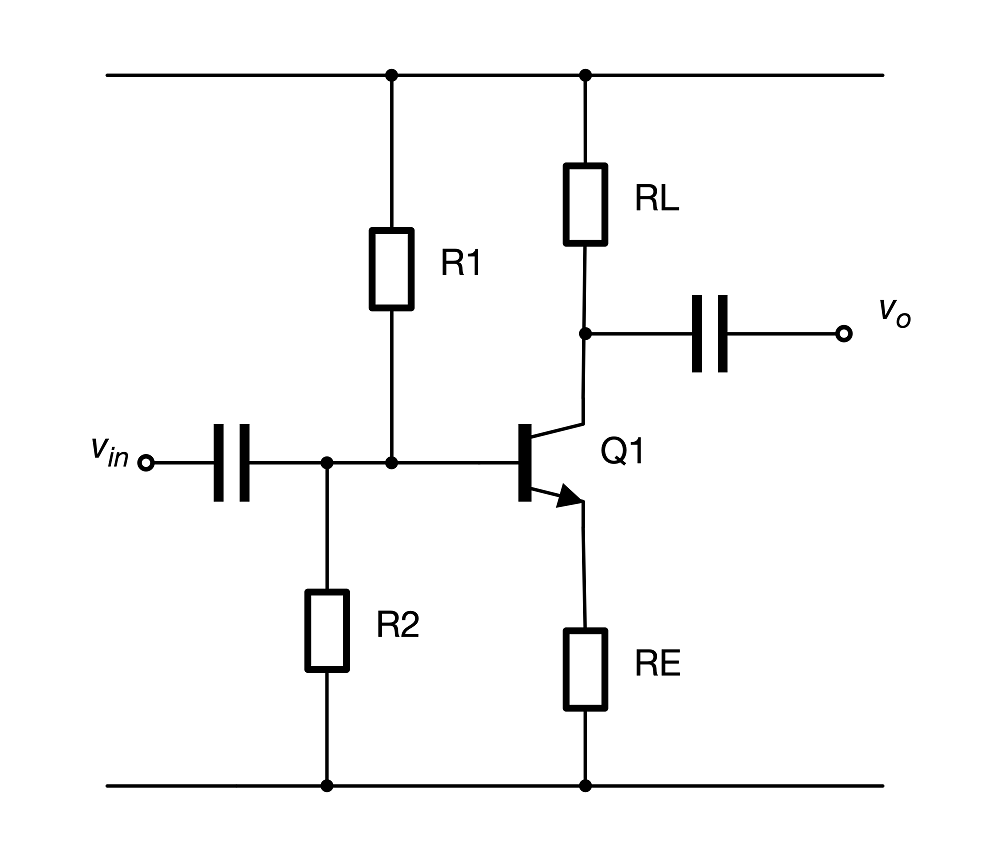# Differential Pairs 101Written by

The differential pair—sometimes called the long-tailed pair—is a widely used building block in electronic circuits, particularly in op amps. The topology pre-dates the solid-state era and is generally attributed to Alan Blumlein, when it appeared in one of his patents in 1936. Its utility stems from its ability to amplify the difference between two input signals while rejecting the part of the signal common to both inputs.FIGURE 1. The classic differential pair is a symmetrical circuit which amplifies the voltage difference between the two inputs and rejects the common-mode voltage. The emitter resistor is often replaced by a current source to improve common-mode rejection and input range.

Figure 1 shows a simple differential amplifier. It is a symmetrical circuit with two inputs and (optionally) two outputs. In order to analyse this circuit, it is useful to take a couple of steps backward. Consider the AC-coupled common emitter amplifier of Figure 2. Transistor Q1 is biased by R1 and R2 to some operating point. An AC signal applied to the input results in a small change to the transistor’s base-emitter voltage which in turn will cause a change in collector current of the transistor. This will be converted to a voltage change at the collector across the load resistor RL. Note that an increase in collector current results in a decrease in collector voltage so the output signal is inverted. The voltage gain of the stage will therefore be –gmRL.FIGURE 2. It’s useful to build up a picture of how a differential pair works step by step. This AC-coupled common-emitter amplifier amplifies and inverts the input signal. The emitter resistor must be smaller than the load resistor, so the emitter voltage remains relatively stable.

The transconductance gm arises from the Hybrid-pi small signal model for BJTs. This models the transistor as transconductance device where the collector current is proportional to the base-emitter voltage. In the common emitter amplifier, we hold the emitter voltage virtually constant (since RE << RL ) and apply the input signal to the base.

Since we are interested in changes to the base-emitter voltage, we could equally hold the base voltage constant and apply the signal to the emitter and thus change the collector current. This configuration is called a common-base amplifier and is shown in Figure 3. This circuit is identical to the common-emitter configuration except we apply the input signal to the transistor’s emitter instead of its base. The gain is now gmRL which is identical to the first example, but opposite in sign.FIGURE 3. This common-base amplifier is identical to the common-emitter version in Figure 2, except the input signal is injected at the emitter of Q1 instead of the base. The gain is the same except it is of the opposite sign. Unfortunately, the input impedance is quite low.

This means we can create a crude differential amplifier with just one transistor. If we consider the base to be the inverting input and the emitter to be the non-inverting input, we can say the output voltage will be (v+ – v)gmRL. Job done!FIGURE 4. Adding an emitter-follower stage addresses the issue of the input impedance of the common-base amplifier of Figure 3. This is starting to look a lot like a differential pair—just remove the ac coupling and bias components and add another load resistor in Q2’s collector and you have the circuit of Figure 1.

However, there is a problem. The input impedance of the inverting input at the base is quite high, whereas it is quite low at the non-inverting input since the emitter resistor is necessarily a low value compared to the load resistor. We can solve this by adding an emitter-follower buffer to the non-inverting input as per Figure 4. Note that there are now two base-emitter junctions back-to-back between the inputs. This is starting to look a lot like a classic differential pair. If we drop the ac coupling, bias resistors and add a second load resistor we have the circuit of Figure 1.

The bias point is established by ensuring that a relatively constant current flows through the emitter resistor. This is done by using a fairly high value resistor or a current source which results in a relatively high voltage across it compared to the input range. This is where the name “long-tailed pair” comes in. With no differential input voltage (in other words, the transistor bases are at the same voltage) the bias current will be split equally between the two transistors. When a differential voltage appears, the current will increase in one transistor and decrease in the other, but their sum will remain constant.

We have already pretty much derived the differential gain of this circuit. Any differential signal applied to the inputs will be split evenly across the two base-emitter junctions, but with opposite signs. Thus, the output of Q1 will be:

… and the output of Q2 will be its inverse:

We could take a differential output voltage across the two collectors:

The common-mode gain is defined by the matching of the two stages and the “stiffness” of the resistor or current source at the emitter of the two transistors. Achieving really good common-mode rejection usually requires the resistor be replaced by an active current source of some kind.

References:

“Alan Blumlein.” In Wikipedia, November 29, 2020. https://en.wikipedia.org/w/index.php?title=Alan_Blumlein&oldid=991374661.

“Hybrid-Pi Model.” In Wikipedia, March 22, 2020. https://en.wikipedia.org/w/index.php?title=Hybrid-pi_model&oldid=946740295

“Chapter 12: Differential Amplifiers [Analog Devices Wiki].” Accessed January 15, 2021. https://wiki.analog.com/university/courses/electronics/text/chapter-12

 Keep up-to-date with our FREE Weekly Newsletter! Don't miss out on upcoming issues of Circuit Cellar. Subscribe to Circuit Cellar Magazine Note: We’ve made the May 2020 issue of Circuit Cellar available as a free sample issue. In it, you’ll find a rich variety of the kinds of articles and information that exemplify a typical issue of the current magazine. Would you like to write for Circuit Cellar? We are always accepting articles/posts from the technical community. Get in touch with us and let's discuss your ideas.